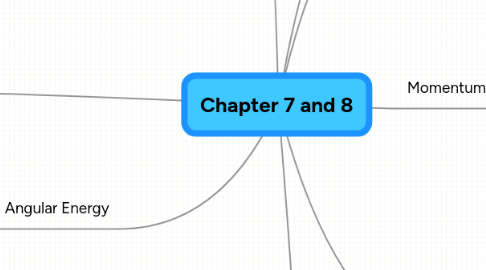# Chapter 7 and 8

Jetzt loslegen. Gratis!Chapter 7 and 8 von## 1. Words of the Day

### 1.1. Applicability

1.1.1. able to apply

1.1.1.1. what areas does energy apply

1.1.2. use for

1.1.2.1. non constant acceleration

1.1.2.2. final velocity

1.1.2.3. changes in position/direction

### 1.2. Trust

1.2.1. firm reliance on the integrity, ability, or character of a person or thing

## 2. Momentum

2.1.1. p = mv

### 2.3. Conservation of Momentum

2.3.1. p = 0

2.3.2. If no external force acts on system, the initial momentum of the system is equal to the final momentum of the system.

## 3. Three types of Collisions

### 3.1. Completely Inelastic

3.1.1. Perfect Sticking

3.1.2. Conservation of only momentum

3.1.3. m1v1i +m2v2i = (m1 +m2 )vf

### 3.2. Inelastic

3.2.1. “Sticky” but the bodies do not stick together

3.2.2. Conservation of momentum only

### 3.3. Elastic

3.3.1. Perfect bouncing

3.3.2. Conservation of kinetic energy and momentum

3.3.3. m1v1i +m2v2i = m1v1f +m2v2f

## 4. System of Particles

### 4.1. Center of Mass

4.1.1. Average location of the mass in a system of particles

4.1.2. 3D in general

### 4.2. Motion of the Center of Mass

4.2.1. Definition of Momentum

4.2.2. Newton’s 2nd Law for Systems

## 5. Angular Energy

### 5.1. Rotational Kinetic Energy

5.1.1. k= 1/2*l*w^2

### 5.2. Moment of inertia

5.2.1. Depends on mass and shape

## 6. Rotational Dynamics

### 6.1. Torque

6.1.1. Angular Force

6.1.2. Vector Quantity

6.1.2.1. T

6.1.3. Magnitude

6.1.3.1. T= -/+ F*D

6.1.3.2. T= F r sin X

6.2.1. F=0

6.2.1.1. Linear

6.2.2. T=0

6.2.2.1. Angular

### 6.4. Rolling Bodies

6.4.1. Add translational and rotational kinetic energies

### 6.5. Refer to page 258, Table 8.1

6.5.1. shapes

6.5.2. axis of rotation

6.5.3. rotational inertia